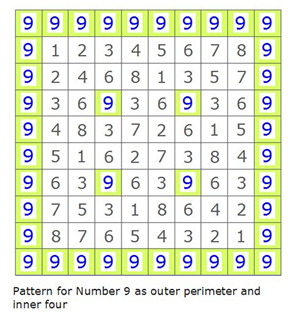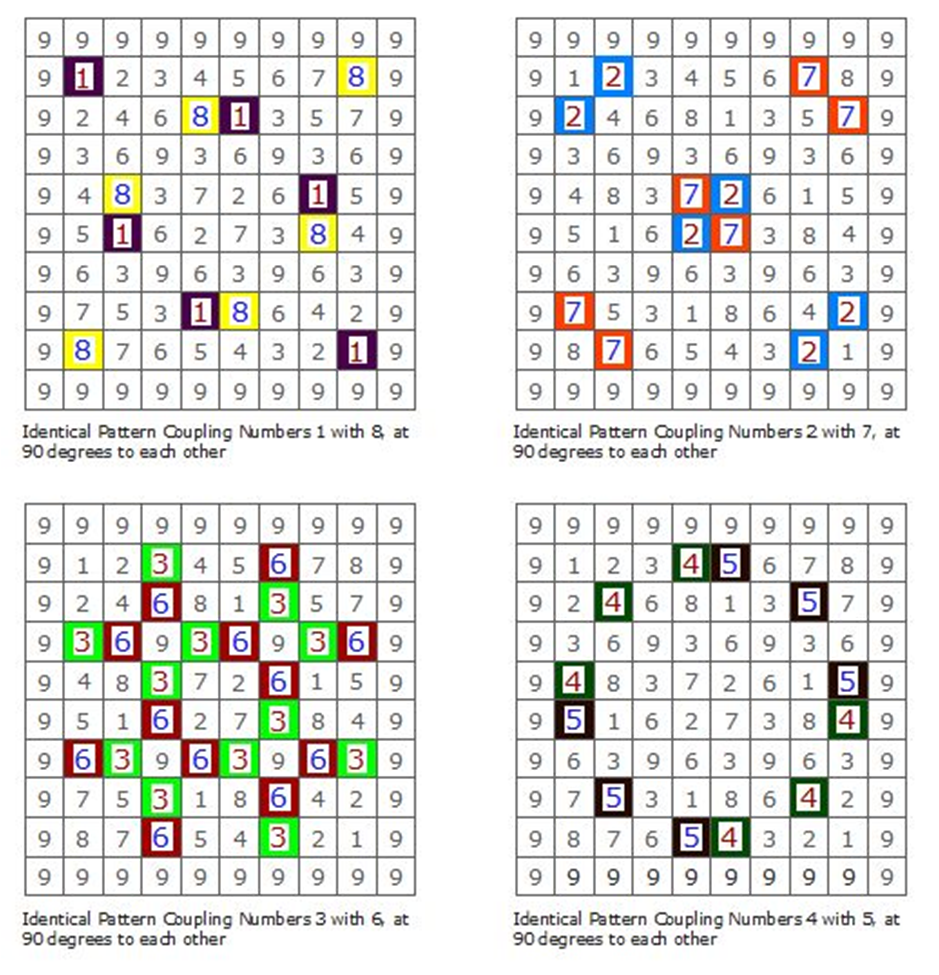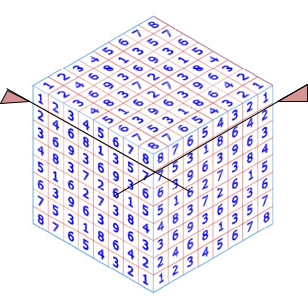# What is discovered – 2/7

### My Discovery

My next deduction or realisation, and my first ‘discovery’, was that the maths of the square works equally from the number 1 in the opposite bottom right hand corner, as well as the top left corner, thus creating a full perimeter of the number 9, around the whole square; i.e. the square is multi-directional!

It can be seen that each number lies in a regular pattern about either diagonal. The regular pattern, created by each number, is identically copied by its opposing twin or ‘coupling’ number, i.e. the following  ‘coupling’  numbers in the square are identical, but are perpendicular (90 degrees) to one another. Each two numbers of the coupling also happen to add up to 9, the relevance of which will be made clear later.

The four number couplings are 1 and 8, 2 and 7, 3 and 6, and 4 and 5. In effect therefore, there are only four basic numbers, or number patterns, each with a mirrored image reflected in its coupling number, and each couple or combinations of number couples adds to 9 i.e. 1 + 8 = 9; 2 + 7 = 9; 3 + 6 = 9; and 4 + 5 = 9. The number 9 is common to all and as I will show, this number is the underlying structure to all, including in the physical form.### My amended ‘Arabic Magic’ Number Square

Refer to Figure 3 below to see the pattern layout of the four number couplings in the amended (from the D Saltman square) two dimensional number square and the number nine as something integral but different; in the 2 dimensions of this number square the number nine hints at a regular structural form within which the four number couplings sit.Fig 3. Pattern layouts of the four number couplings and the structural number nine

### The Next Step toward Three Dimensions

I then wondered whether or not this amended D Saltman ‘Arabic’ Magic Square of numbers (excluding the perimeter nines for clarity) could fit logically on the six sides of a cube. It worked perfectly (see illustration Fig 4), with the number 1 meeting on the three faces of each of four corners of the cube and equally the number 8 meeting on the three faces of the other four corners. The numbers running between the ones and eights, along the cube edges, also matched along both shared faces to the edge. The number nine now becomes the outer shell to the entire cube, but will be left off at this point for clarity.Fig 4. Six copies of my amended two dimensional D Saltman ‘Arabic’ Magic Square, excluding the outer nines for clarity, successfully marry at all eight corners and twelve edges of a cube.

With numbers marrying along the corners and edges of the cube, the next logical step was to consider whether or not there was a hidden potential to be discovered of mathematically related numbers within the cube itself.

My first attempt in 1978 was unsuccessful. I sliced off the top layer of numbers from one face, giving me the edge numbers of the next square layer below, starting with 2 in two of the corners and 7 in the other two corners. Note the correlation with the number couplings mentioned above, 1 and 8 in the corners of the first layer and 2 and 7 in the corners of this next layer below.

When I multiplied the outer edge numbers of this second layer square, to determine the inner numbers, as per the maths that I deduced was used for the first layer (i.e. the ‘Arabic Magic Square), the results were unsuccessful and I believed then, and still do, that inner numbers by this method are probably meaningless. I was disappointed and left this cube as a curiosity, in amongst the sheets I had of other ideas, sketches and concepts.